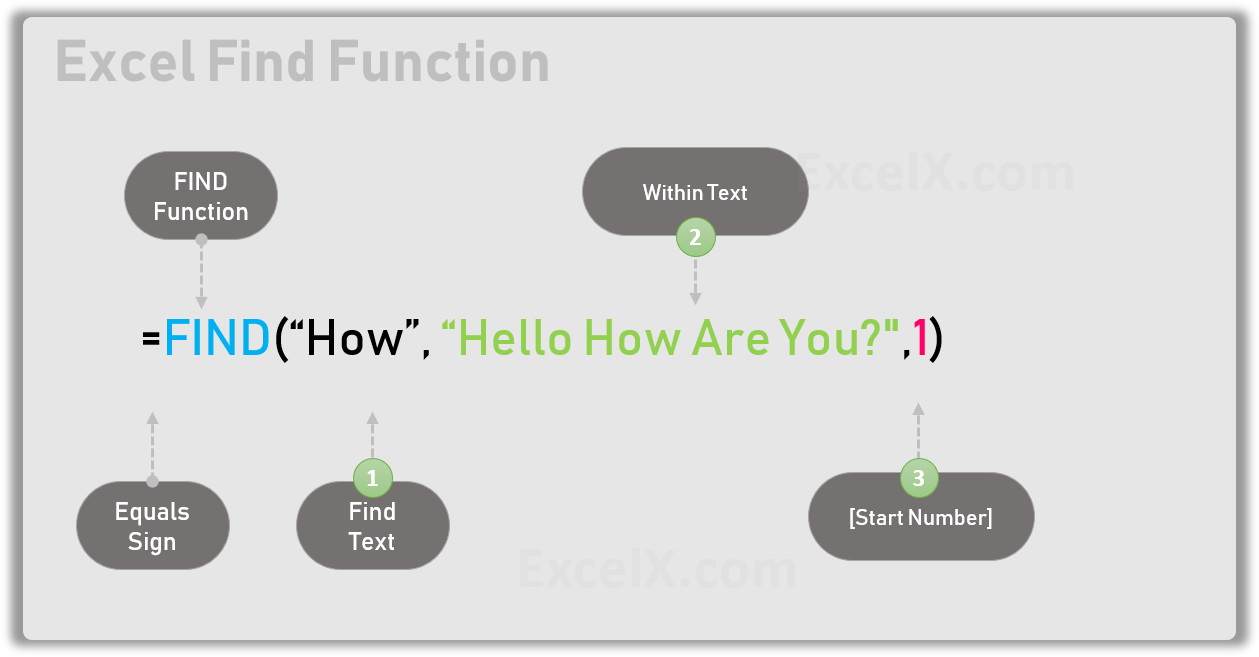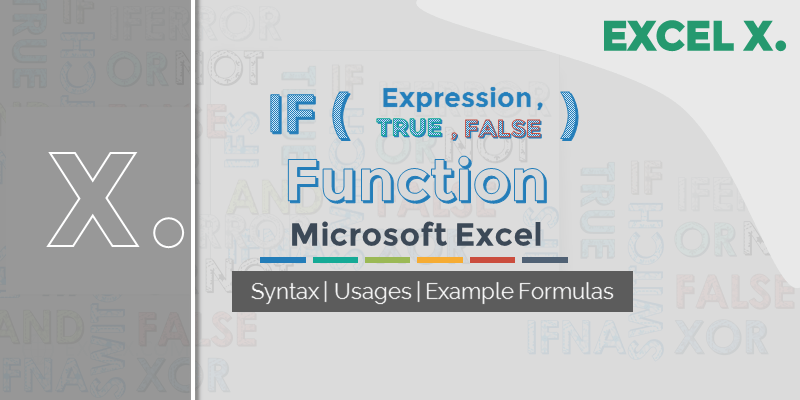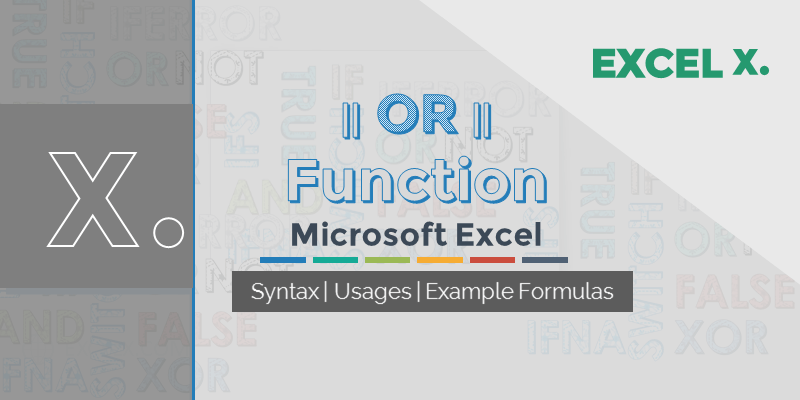# Functions

•Excel Search Function
Gallery

## Excel Search Function

Search Function in Excel searches for a sub-string in another string and returns the position (as a number) of the given sub-string inside another string.

## IFERROR Function in Excel

IFERROR is an Excel Logical Function to Check if a value is an Error. IFERROR used in Excel to handle if the formula is evaluated

## VLOOKUP Function in Excel

VLOOKUP Function in Excel helps to lookup the corresponding values of a Range,Table and Arrays.  VLookup match the given lookup value in the first column

## Function Arguments in Excel

Arguments in Excel Functions are input values to an Excel Function. Most of the Excel Functions will take one or more arguments, will be used

•Excel FIND Function
Gallery

## Excel FIND Function

FIND function in Excel returns the position (as a number) of the given string inside another string. FIND returns a #VALUE error if there is

•IF Function in Excel
Gallery

## IF Function in Excel

IF Function helps to evaluate the logical expression and return the values for both Boolean results. We can specify two values or expressions to return

•NOT Function in Excel
Gallery

## NOT Function in Excel

NOT function in Excel creates Reverse of the given logic. For example, !k=5 means  K5 .

•OR Function in Excel
Gallery

## OR Function in Excel

OR function is very useful to check if any of the condition is True. OR function returns TRUE if any pf the expressions passed as

•AND Function in Excel
Gallery

## AND Function in Excel

AND function is helps to check if all conditions are True. AND function returns TRUE if all the expressions passed as its arguments are true,

•Logical Functions
Gallery

## Logical Functions

Logical Functions in Microsoft Excel helps to check if an expression is True or False based on a logic. For example, we can check if two